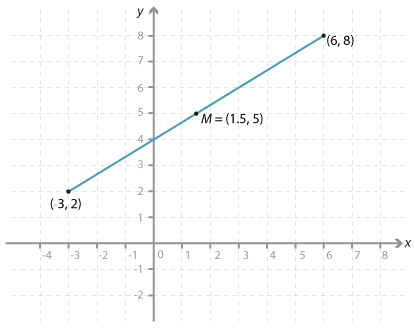### Midpoint of an interval

The midpoint of an interval with endpoints $$P(x_1, y_1)$$ and $$Q(x_2, y_2)$$ has coordinates

$$(\dfrac{x_1 + x_2}{2},\ \dfrac{y_1 + y_2}{2})$$.

The coordinates are found by calculating the average of the x-coordinates and the average of the y-coordinates.

#### Example 3

Find the coordinates of the midpoint of the line interval joining the points (6, 8) and (−3, 2).

#### Solution

The midpoint M has coordinates

$$(\dfrac{6 + (-3)}{2},\ \dfrac{8 + 2}{2}) = (\dfrac{3}{2},\ 5)$$.Detailed description